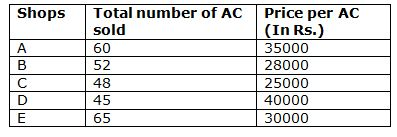# CWC/FCI Prelims 2019 – Quantitative Aptitude Questions (Day-40)

Dear Readers, Exam Race for the Year 2019 has already started, To enrich your preparation here we are providing new series of Practice Questions on Quantitative Aptitude – Section for CWC/FCI Exam. Aspirants, practice these questions on a regular basis to improve your score in aptitude section. Start your effective preparation from the right beginning to get success in upcoming CWC/FCI Exam.

[WpProQuiz 5914]

Directions (Q. 1 – 5): In the following questions, two equations I and II are given. You have to solve both the equations and give answer as,

a) If x > y

b) If x ≥ y

c) If x < y

d) If x ≤ y

e) If x = y or the relation cannot be established

1) I) 5x – 4y = -5

II) 3x + 5y = 34

2) I) 2x2 + x – 66 = 0

II) y2 + 15y + 56 = 0

3) I) x2 + 15x – 76 = 0

II) y2 – 19y + 84 = 0

4) I) 3x2 – 2x – 33 = 0

II) 4y2 + 24y + 36 = 0

5) I) x2 = 156 ÷ 6 + 32 % of 800 + (6 × 7)

II) y = ∜331776

Directions (Q. 6 – 10) Study the following information carefully and answer the given questions:

The following table shows the total number of Air conditioner (AC) sold in 5 different shops in a certain month and the price per AC also given.Note: Total Revenue = Total number of AC sold * Price per AC

6) Total number of AC’s sold in the shop of D is what percentage of total number of AC’s sold in the shop of A and E together?

a) 44 %

b) 36 %

c) 57 %

d) 68 %

e) None of these

7) Find the average price per AC of all the given shops together?

a) 35700

b) 39200

c) 28300

d) 31600

e) None of these

8) Find the ratio between the total revenue of shop B and C together?

a) 91 : 75

b) 85 : 73

c) 77 : 52

d) 115 : 91

e) None of these

9) Find the difference between the total revenue of shop D and shop E?

a) 190000

b) 210000

c) 150000

d) 230000

e) None of these

10) The price per AC in shop C is what percentage of the price per AC in shop D?

a) 74 %

b) 62.5 %

c) 86 %

d) 93.5 %

e) None of these

Direction (1-5) :

5x – 4y = -5 —-> (1)

3x + 5y = 34 —-> (2)

By solving the equation (1) and (2), we get,

X = 3, y = 5

X < y

I) 2x2 + x – 66 = 0

2x2 + 12x – 11x – 66 = 0

2x (x + 6) – 11 (x + 6) = 0

(2x – 11) (x + 6) = 0

X = 11/2, -6 = 5.5, -6

II) y2 + 15y + 56 = 0

(y + 8) (y + 7) = 0

Y = -8, -7

X > y

I) x2 + 15x – 76 = 0

(x + 19) (x – 4) = 0

X = -19, 4

II) y2 – 19y + 84 = 0

(y – 12) (y – 7) = 0

Y = 12, 7

X < y

I) 3x2 – 2x – 33 = 0

3x2 + 9x – 11x – 33 = 0

3x (x + 3) – 11 (x + 3) = 0

(3x – 11) (x + 3) = 0

X = 11/3, -3 = 3.667, -3

II) 4y2 + 24y + 36 = 0

4y2 + 12y + 12y + 36 = 0

4y (y + 3) + 12 (y + 3) = 0

(4y + 12) (y + 3) = 0

Y = -12/4, -3 = -3, -3

X ≥ y

I) x2 = 156 ÷ 6 + 32 % of 800 + (6 × 7)

X2 = (156/6) + (32/100)*800 + 42

X2 = 26 + 256 + 42 = 324

X = 18, -18

II) y = ∜331776

Y = 24

X < y

Direction (6-10) :

Total number of AC’s sold in the shop of D = 45

Total number of AC’s sold in the shop of A and E together

= > 60 + 65 = 125

Required % = (45/125)*100 = 36 %

The total price per AC of all the given shops together

= > 35000 + 28000 + 25000 + 40000 + 30000

= > 158000

Required average= 158000/5 = 31600

Required ratio = [52 * 28000] : [48*25000]

= > 91 : 75

The total revenue of shop D

= > 45*40000 = Rs. 1800000

The total revenue of shop E

= > 65*30000 = Rs. 1950000

Required difference = 1950000 – 1800000 = 150000